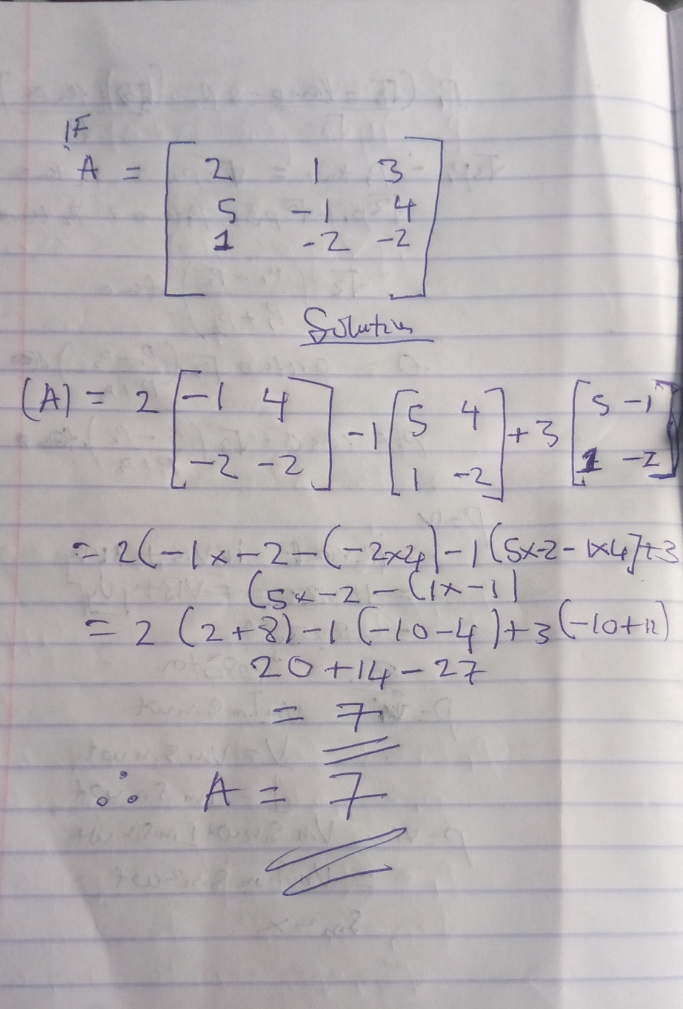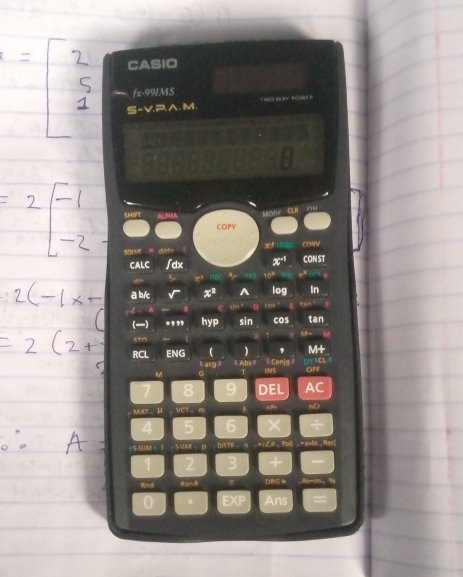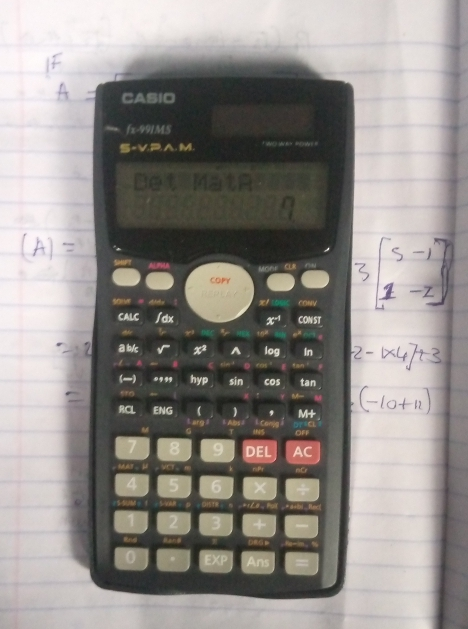# THE FASTEST MEAN OF SOLVING MATRIC EQUATION

in STEMGeeks2 months ago

The fastest mean of solving matric Equation is calculator mean which have been helping me in my school interm of matric topics. it has not only help me but it has equally help my friends.
Before we look at the procedure let's knows what is matric and different types of matric.

What is Matric?

Matric is the rectangular array of real and complex number in row and columns enclose between bracket.
Types of matric.
Line matric: These matric consists only one row.
column matric: it's also one but a column (i.e it consists only one column)
Single element column: it consists of single number in bracket e.g (2) (4) (6)
square matric: A square matric is 2by2 or 3by3 matric.
diagonal matric: These matric have zero entrance everywhere.
Unit matric: in these all the diagonal element are equally to one and it's represented by l.
Scaler matric: This is where all the element are thesame.
Triangler matric. This is the Matric that is split into two triangle with zero entrance on the side of the triangle. This is subdivided into lower and upper triangler.

Example.Okay let's solve using calculator.Procedures.
so we taking it step by step
Note: Anytime you're pressing and you make a mistake you will reset the calculator by pressing "shift and mode" then press 3 to reset.
Step 2: press "mode" three times and select MAT which is 2
Step 3: press "Shift and 4" and press Det which is 1. "copy reply" left hand side in your calculator to move to next which you are selecting.(Because we are looking for Our A which is determinate).
Step 4: press "Shift and 4" again, select Mat which is 3 and select A which is 1.
Step 5: it will deplay Det MatA
step 6: press "Shift and 4" again and select Dim which is 1 and press A1. it will deplay MatA(mxn) m? you will press 3=3 (i.e 3by3 matric if you are looking for 2by2 you insert 2by2).it will deplay mata11.
Step 7: You insert your matric numbers one after the other. The numbers are 2,1,3,5,-1,4,1,-2 and -2 Note: Each time you insert it will deplaying Mata 11,12,13,21,22,23,31,32 and 33 matas.
You press 2 and press "Equal to" in your calculator press 1 and = ,3 and =, 5 and =,-1 and =, 4 and =, 1 and =, -2 and = and -2 and equal to.
and after inserting all the numbers you press AC to clear the numbers.
Step 8: press "Shift and 4" and select Det which 1 ("Copy reply" left hand side in your calculator to move to next)."press Shift and 4"
Step 9 : Select Mat which 3 and press 1 and equal to = and it will deplay 7 as the answer.Note: Anytime you're pressing and you make a mistake you will reset the calculator by pressing shift and mode then press 3 to reset.